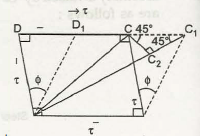To understand the relationship between E and G, take square block ABCD

Shear strain  = ϕ

Strain of AC = AC1 – AC / AC = C1 C2 / AC

= CC1 cos 45° / √2 = CC1 / 2 AB1 /2 (CC1 / AB) = 1/2  (CC1 / BC) = 1/2 ϕ

=  1/2  x t / G

Again, AC has the tensile strain and it takes place due to the stress

σ = σ / E

= t / E

On the diagonal AC the tensile stress takes place because of the compressive stress on BD

= µ . t /E

Combined effect of the two different stresses on the given

Diagonal AC = t / E + µ t /E = t /E (1+µ)

By equating both the equation, we have

t = 2G  = t / E  (1+µ)

G = E / 2 (1 +µ)

where ,

µ = Poisson’s ratio

G = modulus of rigidity

E = modulus of elasticity.

Links of Next Mechanical Engineering Topics:-### Customer Reviews

My Homework Help
Rated 5.0 out of 5 based on 510 customer reviews at